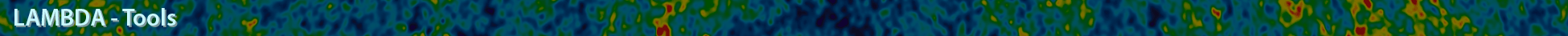# SZ Halo Catalog

```Cosmological parameters are based closely on the five year WMAP data release.

nsinit  = 0.96
hubble0 = 0.71
wde     = -1.0
sigma8  = 0.80
omegam  = 0.264
omegal  = 0.736 = 1-omegam
omegab  = 0.044
YHe     = 0.24
XH      = 0.76
Tcmb    = 2.726

The TPM N-body code was run with 1024^3 particles in a 1000 Mpc/h box. Halos were identified using a
friends-of-friends algorithm and those belonging to a light cone spanning an octant for redshifts 0 < z < 3 have
been saved.

TPM N-body simulation
L  = 1000 Mpc/h
Np = 1024^3
mp = 6.82E10 Msolar/h = 9.61E10 Msolar

FoF halo finder
bfof  = 0.2 times mean interparticle spacing
Nmin  = 293 particles
Mmin  = 2.00E13 Msolar/h = 2.82E13 Msolar
Nhalo = 1414339

SZ maps are available as binary FITS files with the minimum header. Maps are in Healpix format with Nside = 8192
and Npix = 12*Nside^2. Full sky maps are constructed by replicating the octant as follow:

Northern hemisphere
theta_N = theta_O
phi_N   = phi_O + n*pi/2 where n = 0,1,2,3

Southern hemisphere
theta_S = pi - theta_N
phi_S   = 2*pi - phi_N

Sky_sz_nu.fits are distortion maps, in units of Delta T/T, at various GHz frequencies. These maps are for the
redshift range 0 < z < 10. They include relativistic corrections.

Sky_tsz.fits and Sky_ksz.fits are the Compton y and Doppler b maps, respectively. These maps are for the same
redshift range, but do not include relativistic corrections.

Sky_sz_148.fits
Sky_sz_219.fits
Sky_sz_277.fits
Sky_sz_30.fits
Sky_sz_90.fits
Sky_sz_350.fits
Sky_tsz.fits
Sky_ksz.fits

SZ halo catalogs are available in ascii and binary formats. There are 1414339 halos in the first octant for
redshifts 0 < z < 3. For each halo, there are 48 numbers as described below.

Here is a sample codelet to read in the binary data file

integer(4), parameter           :: nhalo = 1414339
real(4),    dimension(48,nhalo) :: halo

open(1,file='halo_sz.binary',form='binary')
do i=1,nhalo
enddo
close(1)

Halo array
halo(    1,i) = redshift
halo(    2,i) = ra  in degrees
halo(    3,i) = dec in degrees
halo(  4:6,i) = comoving position of halo potential minimum in Mpc
halo(  7:9,i) = proper peculiar velocity in km/s
halo(   10,i) = Mfof in Msolar

halo(   11,i) = Mvir     in Msolar
halo(   12,i) = Mgas_vir in Msolar
halo(   13,i) = Rvir     in proper Mpc
halo(   14,i) = Integrated TSZ within Rvir in arcminute^2
halo(   15,i) = Integrated KSZ within Rvir in arcminute^2
halo(16:21,i) = Integrated SZ at 148,219,277,30,90,350 GHz

halo(22:32,i) = Same as 11-21 but for within R200
halo(33:43,i) = Same as 11-21 but for within R500

halo(   44,i) = Mstar               in Msolar
halo(   45,i) = central gas density in Msolar/Mpc^3
halo(   46,i) = central temperature in keV
halo(   47,i) = central pressure    in Msolar/Mpc/Gyr^2
halo(   48,i) = central potential   in (Mpc/Gyr)^2

```
A service of the HEASARC and of the Astrophysics Science Division at NASA/GSFCHEASARC Director: Dr. Alan P. Smale
LAMBDA Director: Dr. Eric R. Switzer
NASA Official: Dr. Eric R. Switzer
Web Curator: Mr. Michael R. Greason